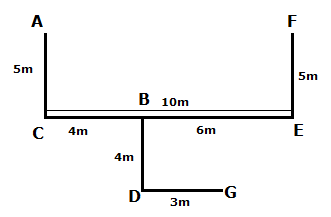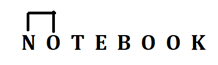# RRB PO Prelims Reasoning Ability Questions 2021 (Day-10)

Dear Aspirants, Our IBPS Guide team is providing new series of Reasoning Questions for RRB PO Prelims 2021 so the aspirants can practice it on a daily basis. These questions are framed by our skilled experts after understanding your needs thoroughly. Aspirants can practice these new series questions daily to familiarize with the exact exam pattern and make your preparation effective.

Start Quiz

Inequality

Direction (1-3): In each of the following questions, the relationship between different elements is shown in the statements followed by two conclusions. Find the conclusion which is definitely true.

a)If only conclusion I follows.

b)If only conclusion II follows.

c)If either conclusion I or II follows.

d)If neither conclusion I nor II follows.

e)If both conclusions I and II follow.

1) Statements:

M ≥ D = J > L < G

Conclusions:

I). M > G

II). L < M

2) Statements:

A ≥ I = K > S ≤ L < O

Conclusions:

I). A > S

II). S < O

3) Statements:

I < Z = U ≥ T > P

Conclusions:

I). Z < P

II). I < T

Direction sense

Direction (4-5): Study the following information carefully and answer the below questions.

Point A is 5m north of point C. Point E is 5m south of point F. Point G is southwest of point E. Point B is 4m north of point D, which is 3m west of point G. Point E is 6m east of point B which is 4m east of point C.

4) In which direction is point E with respect to point A?

A.North-west

B.South-east

C.North-east

D.South-west

E.None of these

5) Four of the following are alike in a certain way so form a group, which of the following one does not belong to that group?

A.AF

B.CE

C.DG

D.BF

E.BE

Alpha numeric series

Direction (6-9): Study the following arrangement carefully and answer the questions given below.

U 1 & N 8 3 7 O % 3 P \$ * A @ 7 ^ T \$ 8 T B % F 7 4 #

6) How many such symbols are there in the above arrangement each of which is immediately preceded by a vowel and immediately followed by a number?

A.1

B.3

C.More than three

D.2

E.None

7) How many such numbers are there in the above arrangement each of which is immediately followed by a number and immediately preceded by a consonant?

A.1

B.None

C.3

D.More than three

E.2

8) If all the symbols are dropped from the given arrangement, then which of the following element is 7th from the right end?

A.T

B.F

C.P

D.B

E.None of these

9) Which of the following element is seventh to the right of sixth element from the left end?

A.P

B.*

C.3

D.%

E.None of these

Misc

10) How many such pairs of letters are there in the word ‘NOTEBOOK’ each of which has as many letters between them as they have in the English alphabetical series (both forward and backward direction)?

A.2

B.3

C.None

D.1

E.More than three

I). M > G      (M ≥ D = J > L < G) => False

II). L < M        (M ≥ D = J > L < G) => True

I). A > S          (A ≥ I = K > S ≤ L < O) => True

II). S < O        (A ≥ I = K > S ≤ L < O) => True

I). Z < P          (I < Z = U ≥ T > P) => False

II). I < T          (I < Z = U ≥ T > P) => False

Directions (4-5) :O % 3, A @ 7

N 8 3, F 7 4